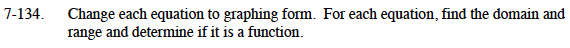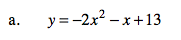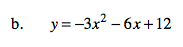Home > CCA2 > Chapter 7 > Lesson 7.2.2 > Problem7-134

7-134.
1. Change each equation to graphing form. For each equation, find the domain and range and determine if it is a function. Homework Help ✎

1. y = −2x2x + 13

2. y = −3x2 − 6x + 12Factor the terms containing x so the coefficient of x² is 1, then complete the square.

$y=-2\left(x^{2}+\frac{1}{2}x\right)+13$

$y=-2\left(x^{2}+\frac{1}{2}x+\frac{1}{16}\right)-(-2)\left(\frac{1}{16}\right)+13$

$y=-2\left(x+\frac{1}{4}\right)^{2}+\frac{105}{8}$See part (a).